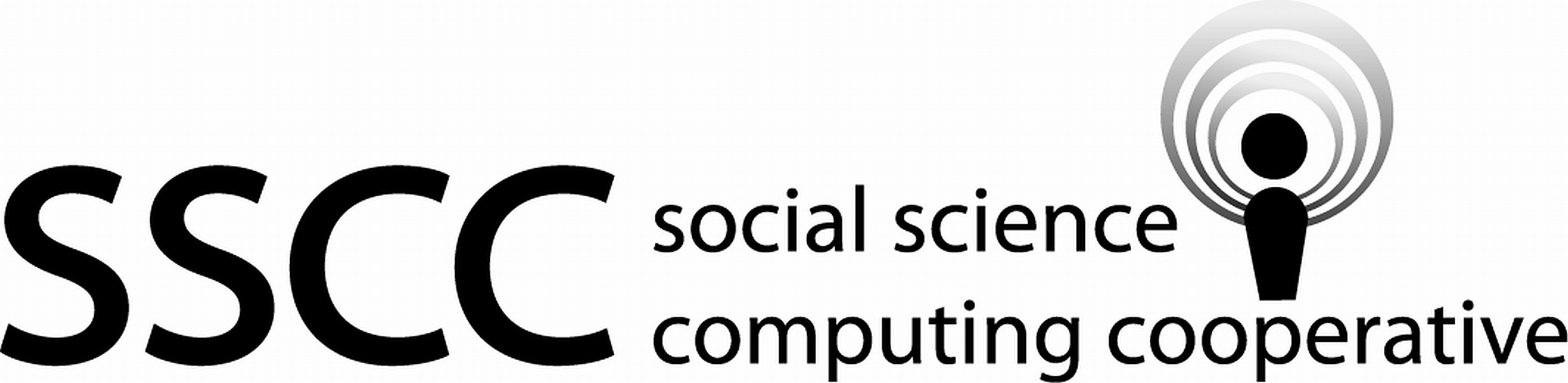Supporting Statistical Analysis for Research

## 2.11 Reshaping data

The gather() method is used to transform a set of columns into two columns. (This is also call going from wide to long.) One of the new columns is the value column. This is a column that contains all of the values that were in the gathered set of columns. The other is the key, a column that contains the name of the gathered column the value came from. The key column is a categorical variable, with a level for each of the gathered variables.

The spread() function is the opposite of gather(). (This is also call going from long to wide.) It takes two columns and transforms them to a set of variables. The names of the new variables will be taken from the levels of the key variable. The values of the new variables comes from the value variable.

### 2.11.1 Examples

1. Converting data to long form

In this example we will gather the three earnings columns in cps into a year and earnings column.

The separate() function is used to separate the numeric year part of the string from real and earn in the year variable.

cps2 <-
cps %>%
gather(
key = year,
value = real_earn,
real_earn_74,
real_earn_75,
real_earn_78
) %>%
separate(year, into = c("X1", "X2", "year"), sep = "_") %>%
select(-X1, -X2) %>%
arrange(id, year)

cps2 %>%
select(id, year,  age, educ, marr, real_earn) %>%
head()
# A tibble: 6 x 6
id year    age  educ  marr real_earn
<dbl> <chr> <dbl> <dbl> <dbl>     <dbl>
1     1 74       45    11     1    21517.
2     1 75       45    11     1    25244.
3     1 78       45    11     1    25565.
4     2 74       21    14     0     3176.
5     2 75       21    14     0     5853.
6     2 78       21    14     0    13496.

Note, the arrange() function sorts a tibble on the variables listed as parameters.

2. Converting data to wide form

In this example we will spread the mean_earn_78 column in cps_eth_marr_earn into columns for marital status.

cps_eth_marr_earn <-
cps_eth_marr_earn %>%
cps_eth_marr_earn
# A tibble: 3 x 3
ethnicity         0    1
3 hisp           10138. 14607.